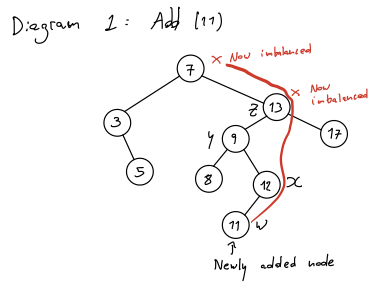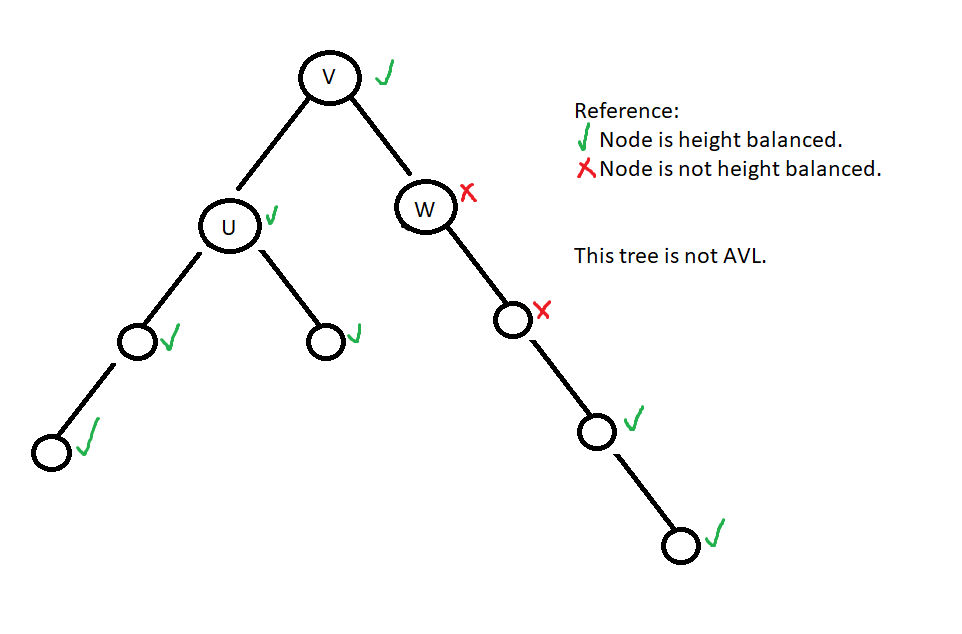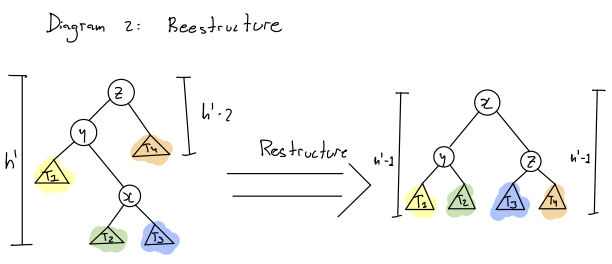Scribes:

• Andy Arrigoni Perez
• Sawyer Pollard
• Luxin Sun
• Cesaire Mugishawayo

Overview:

1. Defining height balance
2. Benefits of balance
3. Maintaining balance efficiency
• Remove

Section 1: Defining height balance

Goal: Since find, add and remove run at O(h) (Where h is the height of the tree, we want to support the add/remove methods to maintain h as small as we can.Definition of height:

Let $$v$$ be a node in a binary tree.

Then, $$h(v)= height$$ (Distance to the most distant descendant leaf)

Note: Convention is that $$h(null) = -1$$

Observation:

If $$u = v.left$$ and $$w = v.right$$,

then $$h(v) = 1 + max(h(u), h(w))$$

Definition: Node $$v$$ is height balanced if the heights of $$v$$’s children differ at most by 1. Formally: $$\lvert h(u) - h(w) \rvert \leq 1$$

Importantly, a binary tree T is (height) balanced or AVL (named for its creators Georgy Adelson-Velsky and Evgenii Landis) if all nodes in T are height balanced.

Example:Section 2: Benefits of Balance

Goal: If T is balanced (or AVL), then its height $$h$$ is $$O(log(n))$$ where $$n$$ is the number of nodes in the tree.

Roundabout Method: Consider $$m(h) = \text{minimum number of nodes in AVL tree of height h}$$

If $$n \leq m(h)$$, then the height of the tree is at most $$h$$.

What is $$m(h)$$ for small values? m(0)=1 | $$m(h)$$ values for small $$h$$ | |–| | $$m(0) = 1$$ | | $$m(1) = 2$$ | | $$m(2) = 4$$ | | $$m(3) = 7$$ | | $$m(4) = 12$$ | | $$m(5) = 20$$ |

What can we say about the structure of an AVL tree of height $$h$$ with a minimal number of nodes? (when height of the tree is at least 2)

Firstly,

$m(h) = 1 + m(h - 1) + m(h - 2)$

(Note: $$m(h - 1) > m(h - 2)$$)

So,

$m(h) > 2 * m(h - 2 > 4*m(h-4) > 8*m(h-6) > \ldots$

This pattern can be generalized to: $$m(h) > 2^i * m(h - 2i)$$

If, $$i=\frac{h}{2} - 1$$

(Note: “round up” $$\frac{h}{2}$$ to the nearest integer.)

Then, $$h-2i$$ can only be $$0$$ or $$1$$.

So,

$m(h - 2i)= m(0) \text{ or } m(1) = 1 \text{ or } 2$ $m(h) \leq 2^i * m(0 \text{ or } 1)$ $m(h) \leq 2^{\frac{h}{2} - 1}$

Taking $$log$$ of both sides:

$log(m(h)) \geq \frac{h}{2} - 1$ $2 * log(m(h)) + 2 \geq h$

Conclusion: If T is an AVL tree with $$n$$ nodes and height $$h$$, then:

$2 * log(m(h)) + 2$ $h \leq 2 * log(n) + 2$

$$h = O(log(n))$$

Section 3: Maintaining BalanceWhen can imbalance occur?

• Only at an ancestor of a newly added node.
• Only in at most $$h$$ nodes, since the tree was previously balanced, and the imbalance only occurs at the ancestors of the newly added node.

Let:

$W = \text{newly added node causing an imbalance (11 in our example)}$ $Z = \text{deepest node where imbalance occurs (13 in our example)}$ $Y = \text{child of z in the direction of W (9 in our example)}$ $X = \text{child of y in the direction of W (12 in our example)}$

Because of the properties of BSTs, we know that: $$Y < X < Z$$

What we want: (Picture of restructure)